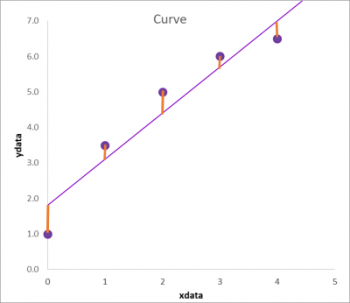# How Least Squares regression works

### Plotting data for curve fit

When you have measured data points in an assay you can plot these in a graph for example in Excel. The x value are values you know, like concentration values of the calibrators. You can then plot your measured values as y values. This can be absorbance, light units or whatever measurement parameter you have.

### Line of best fit

Curve fitting can be used to fit the line of best fit to these points. The line of best fit can be a straight line with function y= A + (B*x) . Alternatively non-linear functions can be used which follow the same method. From the straight line drawn, there is a distance of each actual point to the line. Since we know that our x-values are accurate, the distance of the data point to the line is only caused theoretically be inaccuracy of measurement of our y values. We can then calculate the distance of each y point to the fitted line. This is called a residual. The line of best fit is the line with minimal distance to the measured points. This is a line in which the total distance of the line to the different points is minimal. In practice we use the square of the residuals and add these up. This is called the sum of squares. Since we are using the minimal of the sum of squares to predict the line of best fit this method is called the least squares method.The least squares method uses the distance from the data points to the line of best fit

### Curve fitting iterations

A curve fitting program will not calculate the values of the parameters, in this case A and B of the function y = A + (B*x), but it will try many values for A and B to find the optimal value. The best value for A and B is found with the least squares method when the sum of squares is minimal. A curve fitting program will iterate and use many values for A and B, but it needs values for A and B to start. These are called the start values. If the values for A and B are far away from the optimal value, sometimes the fitting program cannot find the appropriate values as it is impossible to test all possible values. Usually the fitting algorithm only modifies the parameters A and B slightly and then calculates if the modification is better or worse compared to the already calculated values. A great example how a an interation over parameters can influence the residuals can be found on desmos.

### Least squares method excel

You can calculate the values of the residuals manually in excel or you can use Assayfit Pro or another curve fitting program to do it. Assayfit Pro will calculate the best values for the parameters without the need of providing start values as the program will calculate these. It will also plot the line of best fit in excel so you can see if the optimization has been successful.

### Calculating unknowns

The goal of fitting data to a function is to predict unknown measurement values. The y values (absorbance or light units) we measure and would like to known what the corresponding x value (concentration) is. With the inverse of the function we can accurately calculate the value of x if we provide an y value and if the parameters (in this case A and B) are known. In practice we use the y value and read the x value from the curve.

### Weights

Sometimes each data point is not equally important in the determination of the line of best fit. In many cases you would like to fit the line better to the smaller numbers compared to the larger numbers. For assays this is very important for obtaining good sensitivity and precision. A simple example will demonstrate why. If the residual at concentration of 1 is 1 this is relatively a very large deviation as the deviation is as large as the value itself. However If the value is 1000, a deviation of 1 is relatively small. Because of this, it is preferred to have a better fit at a value of 1 (smaller number) compared to 1000. Introducing a fit with a preference for certain data points can be performed by applying weights. Weights are values of relative importance. If you do not apply weights, all data points have a weight of 1. Weights are used to multiply the square of the residual. In the figure above we showed the residuals as orange bars. We first square each orange bar and then multiply each square with the weight. These are then added up to form the weighted sum of squares. Since some values have been multiplied with a high number its residual will be more important in the total sum of squares. A very common approach is to use the value of 1/Y2 as the weight. The value of 1/Y2 (or 1/ absorbance ^2) is much higher with smaller numbers compared to larger numbers. Sometimes 1/standard deviation^2 is a good predictor. If you have multiple measurements of the same point a standard deviation can be determined. Using this as a weight predictor (1/ SD^2) will give more importance to the points with a good (=low) standard deviation.